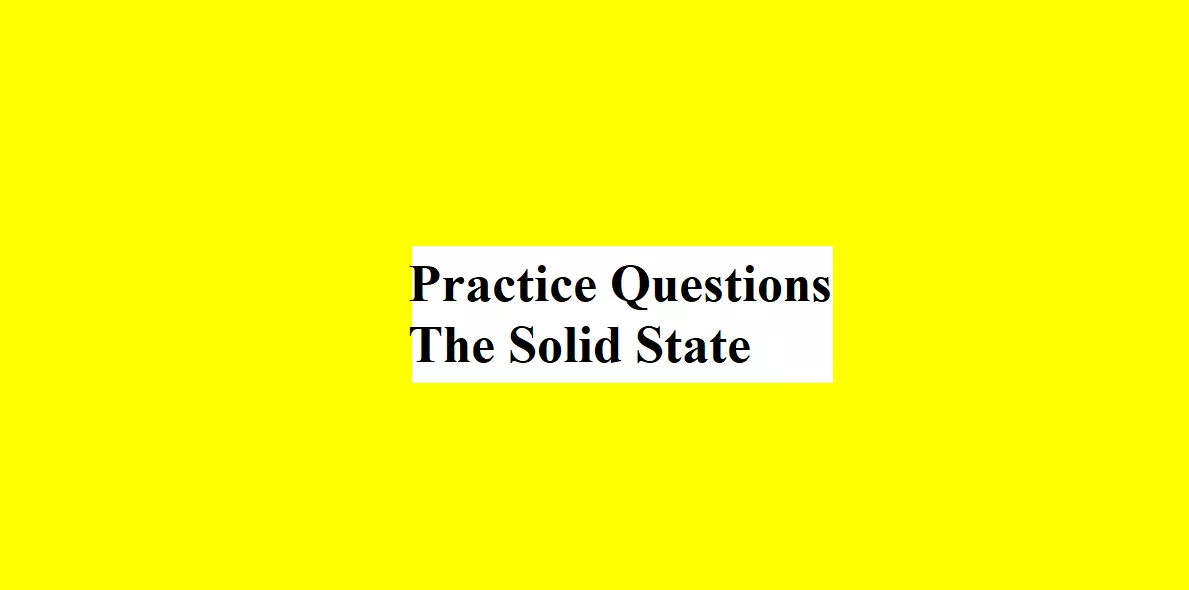# Practice Questions The Solid StatePractice Questions The Solid State

The Solid State

Que 1. Write the name of a crystal showing both Schottky and Frenkel defects.

Que 2. Which of the following lattices has the highest packing efficiency? Explain your answer: (a) Simple Cubic    (b) Body Centred Cubic         (c) Face Centred Cubic

Que 3. The smallest repeating unit of the space lattice is _______

Que 4. A compound is formed by two elements P and Q. Atoms of ‘Q’ (anion) make hcp lattice and those of ‘P’ (cation) occupy all the tetrahedral voids. What is the formula of the compound?

Practice Questions The Solid State

Que 5. Write any four differences between crystalline solids and amorphous solids.

Que 6. ZnO is white in colour but become yellow upon heating. Why?

Que 7. Sodium crystallises in the body-centred cubic unit cell with edge length 4.29A0. What is the radius of the sodium atom?

Que 8. The structure of a mixed oxide is ccp. The cubic unit cell of mixed oxide is composed of oxide ions. One-Fourth of tetrahedral voids are occupied by divalent metal A and all the octahedral voids are occupied by monovalent metal B. Find the formula of oxide.

Que 9. How many unit cells are present in a cube-shaped ideal crystal of NaCl of mass 1 g?

Que 10. A metal crystallises into two cubic phases – fcc and bcc whose unit cell edge lengths are 3.5A0 and 3A0 respectively. Calculate the ratio of the densities of fcc and bcc.

Practice Questions The Solid State

Que 11. Schottky and Frenkel’s defects are stoichiometric defects.
(a) Write any two differences between Schottky and Frenkel defects.
(b) Explain the pink colour of LiCl when heated in the vapours of Lithium.
(c) Why LiCl does not exhibit Frenkel defect?

Que 12. Solids can be classified into three types on the basis of their electrical conductivities.
(a) Name the three types of solids and write their conduction mechanism.
(b) Non-stoichiometric Cuprous oxide (Cu2O) can be prepared in the laboratory. In this Oxide, the Copper to Oxygen ratio is slightly less than 2:1. Can you account for the fact that this substance is a p-type semiconductor?

Practice Questions The Solid State

Que 13. A close-packed structure of uniform spheres has the cell edge = 0.8 mm. Find the radius of molecules if it has:
(1) Simple cubic lattice
(2) BCC lattice
(3) FCC lattice

Que 14. In a close-packed structure of mixed oxides, the lattice is composed of oxide ions. One-eighth of tetrahedral voids are occupied by divalent cations while one-half of octahedral voids are occupied by trivalent cations. What is the formula of the oxide?

Que 15. Define and Explain the radius ratio.

Practice Questions The Solid State